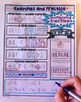# Multiplying Fractions Doodle NotesSubject
Resource Type
File Type
PDF (2 MB|3 plus answer keys & info)
Standards
\$4.25
• Product Description
• Standards
Fraction Multiplication: "doodle notes" -

When students color or doodle in math class, it activates both hemispheres of the brain at the same time. There are proven benefits of this cross-lateral brain activity:
- new learning
- relaxation (less math anxiety)
- visual connections
- better memory & retention of the content!

Students fill in the sheets, answer the questions, and color, doodle or embellish. Then, they can use it as a study guide later on.

Content includes:
- Fraction times a whole number
- Multiplying two fractions
- Formatting Answers (simplest form, mixed numbers as necessary)
- Using a dot instead of x to represent multiplication
- Canceling before multiplying
- Procedures plus visual models and concepts
- Multiplying three fractions
- Multiplying mixed numbers

** NOTE: This resource is also available as part of a DISCOUNTED bundle: Fractions, Decimals, & Percents Activity Bundle

Visual note taking strategies like sketch notes or doodle notes are based on dual coding theory.  When we can blend the text input with graphic/visual input, the student brain processes the information differently and can more easily convert the new learning into long-term memory.

This strategy also integrates the left and right hemispheres of the brain to increase focus, learning, and retention!

You might also like:

Fraction Operations - Golf Game

Fractions: "Choose Your Own Journey" Book

Middle School Pre-Algebra Super Bundle

Interpret the product (𝘢/𝘣) × 𝘲 as a parts of a partition of 𝘲 into 𝘣 equal parts; equivalently, as the result of a sequence of operations 𝘢 × 𝘲 ÷ 𝘣. For example, use a visual fraction model to show (2/3) × 4 = 8/3, and create a story context for this equation. Do the same with (2/3) × (4/5) = 8/15. (In general, (𝘢/𝘣) × (𝘤/𝘥) = 𝘢𝘤/𝘣𝘥.)
Apply and extend previous understandings of multiplication to multiply a fraction or whole number by a fraction.
Total Pages
3 plus answer keys & info